•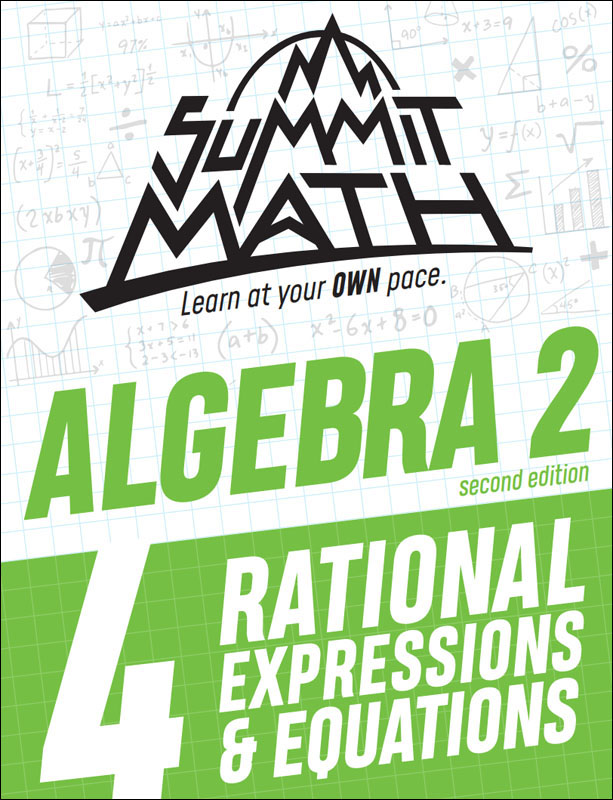•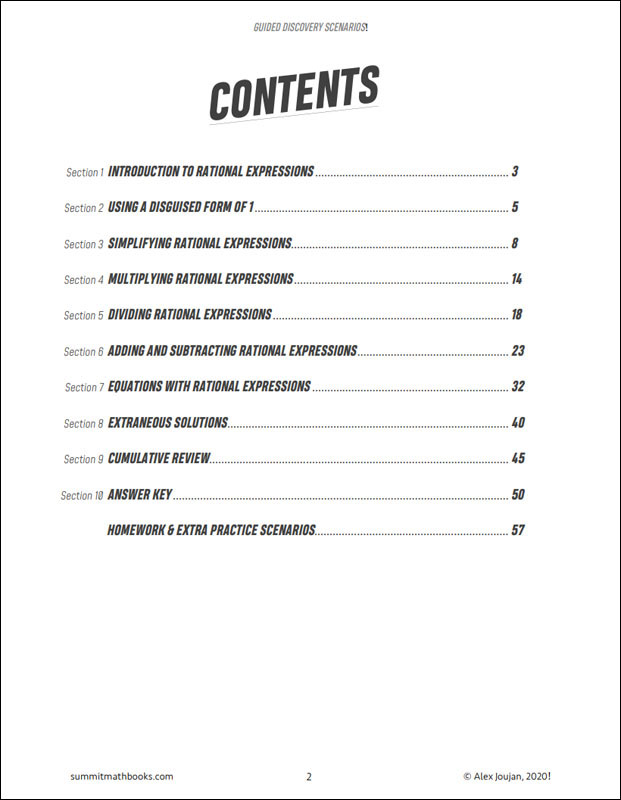•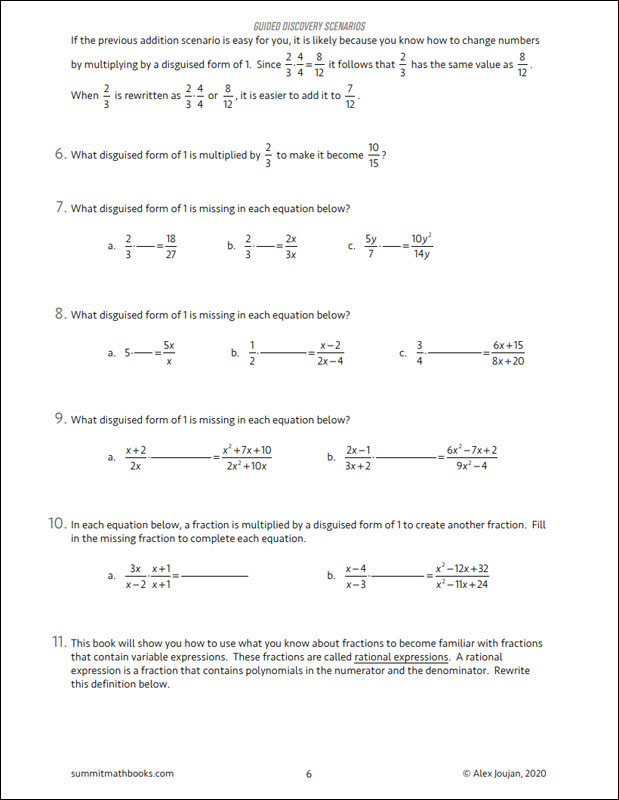•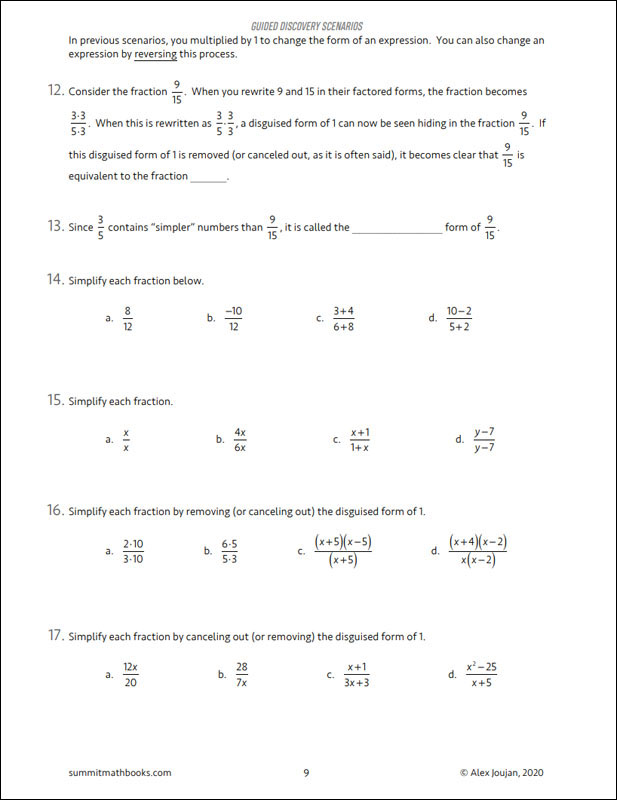•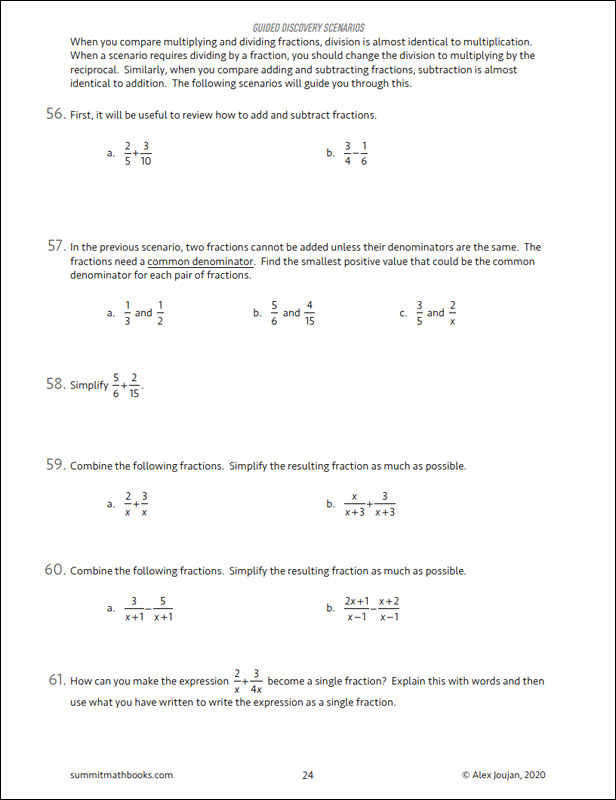# Summit Math Algebra 2 Book 4: Rational Equations & Expressions (2nd Edition)

# 078923

Our Price: \$12.25
Retail: \$12.50
Save: 2.00% (\$0.25)
In Stock.
Qty:
Qty:

Item #: 078923 9781710918403 7-11

#### Publisher Description:

In this book, you will learn how to manipulate a rational expression by multiplying it by a disguised form of 1. You will use this skill to do the following operations with rational expressions: addition, subtraction, multiplication and division. You will then learn how to solve rational equations by identifying common denominators and eliminating the fractions. Near the end of the book, you will learn that some equations have extraneous solutions. This book builds on Algebra 1: Book 5 and Algebra 2: Book 3.

Topics in this book:

• Introduction to rational expressions
• Using a disguised form of 1
• Simplifying rational expressions
• Multiplying rational expressions
• Dividing rational expressions
• Adding and subtracting rational expressions
• Equations with rational expressions
• Extraneous solutions
• Cumulative Review

Category Description for Summit Math Algebra:

If you are searching for an independent algebra program or a supplement to teach specific algebra concepts, Summit Math is worth your consideration. Using the guided discovery method, students make their own connections as they learn to master each scenario before moving on to the next. Each section builds on previous concepts already learned. The first half of the book incorporates the guided discovery approach, while the latter half uses extra practice sheets to improve understanding of the topics. Each section has an answer key. A placement test and pacing guide is provided for each level. The author states this is a complete program teaching typical topics in the Algebra I and II sequence while preparing students for standardized testing. If students feel they need extra instruction, a video subscription is available from the publisher. Free downloadable tests and a more detailed version of the answer key are also available at the publisher’s website.

Primary Subject
Mathematics
7
11
ISBN
9781710918403
Format
Paperback
Brand Name
Summit Math
Weight
0.7 (lbs.)
Dimensions
11.0" x 8.5" x 0.25"